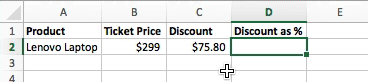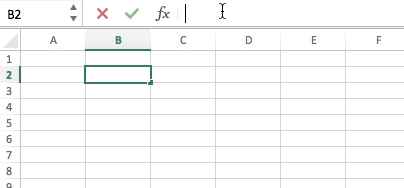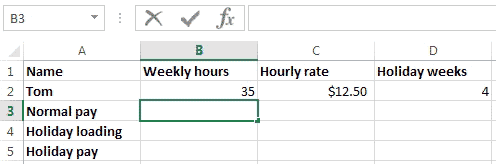### Excel formula

#### Discount as percentage | # gomaths.net/4784    User submitted, thanks to Simon Job

Calculate discount as a percentage from sale price and discount amount.

`=C2/B2`#### Generate Random Integers | # gomaths.net/4247    User submitted, thanks to Simon Job

Use the RANDBETWEEN function to generate random integers.

`=RANDBETWEEN(bottom,top)`

where bottom is the smallest integer RANDBETWEEN will return and
top is the largest integer RANDBETWEEN will return.

For example, to simulate a six-sided dice.

`=RANDBETWEEN(1,6)`#### Holiday Pay | # gomaths.net/4220    User submitted, thanks to Simon Job

Calculate the holiday pay.

`=0.175*B3`

where `B3` is 4 weeks normal pay